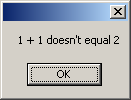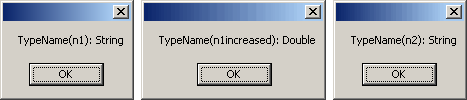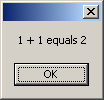### QTP VBScript - Are you sure 1+1=2?

QTP VBScript contains different niceties. This article shows that 1 + 1 doesn't equal 2 sometimes.

 Exactly, 1 + 1 doesn't equal 2 sometimes.

Well, I've prepared this QTP script:
n1 = InputBox("Enter first number")
n2 = InputBox("Enter second number")
n1increased = n1 + 1

If n1increased = n2 Then
MsgBox n1 & " + 1 equals " & n2
Else
MsgBox n1 & " + 1 doesn't equal " & n2
End If

I will explain it step by step and show all entered data and generated result.
Read first number from user's input
I enter 1 as a first number:

Read second number from user's input
I enter 2 as a second number:

1. Increase first number by 1
n1increased = n1 + 1
2. Check whether first increased number equals to second number
If n1increased = n2 Then
MsgBox n1 & " + 1 equals " & n2
Else
MsgBox n1 & " + 1 doesn't equal " & n2
End If
And the result is:Do you know why it is so? What is a reason?
The answer is simple. This is due to converting of VBScript types.

After you read first number (1) from user and assign it (value of course value, not user :) ) to variable, this variable has value of String type.
So, this is the same like n1 = "1"

When VBScript executes this line:
n1increased = n1 + 1
it sees, that we perform mathematical operation and converts n1 into number.
Then VBScript calculates n1 + 1 and the result (2) has a numeric type - Double (special double-precision floating-point value).

Here is the main point! 2 as Number is not the same as 2 as String. These values have different type and that's why they differ.
Simple visual example - two apples are not equal to two pears :)

I can demonstrate the above types converting.
I've added this code into our QTP script before if-then-else:
MsgBox "TypeName(n1): " & TypeName(n1)
MsgBox "TypeName(n1increased): " & TypeName(n1increased)
MsgBox "TypeName(n2): " & TypeName(n2)
And its result is:That's why the value of number variable is not equal to the value of string variable.

Well, How to make that 1 + 1 = 2 ?
Answer: Convert string value to number with CInt function:
If n1increased = CInt(n2) Then
MsgBox n1 & " + 1 equals " & n2
Else
MsgBox n1 & " + 1 doesn't equal " & n2
End If
And the result is:It works. It works correctly :)

I hope, you will keep in mind this issue with VBScript types converting. It can save your time during debugging of QTP scripts.

#### 1 comment:Anonymous said...

awesome bro,..